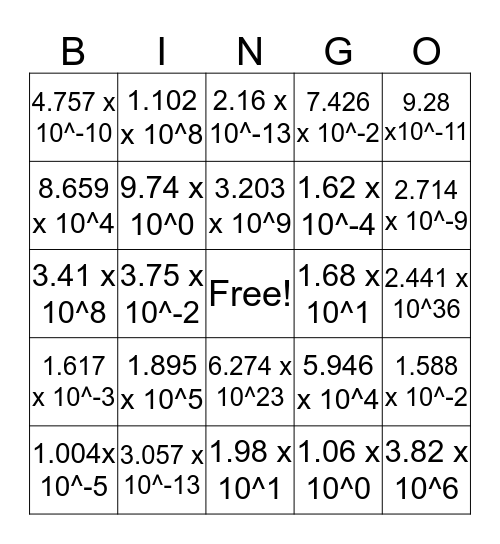# Untitled BingoThis bingo card has a free space and 24 words: 1.895 x 10^5, 3.057 x 10^-13, 3.203 x 10^9, 1.004x 10^-5, 9.28 x10^-11, 4.757 x 10^-10, 8.659 x 10^4, 1.102 x 10^8, 1.68 x 10^1, 6.274 x 10^23, 1.62 x 10^-4, 3.41 x 10^8, 5.946 x 10^4, 1.588 x 10^-2, 9.74 x 10^0, 1.06 x 10^0, 2.441 x 10^36, 2.16 x 10^-13, 2.714 x 10^-9, 3.75 x 10^-2, 3.82 x 10^6, 1.617 x 10^-3, 7.426 x 10^-2 and 1.98 x 10^1.

## Play Online